### 5.1.3 Basic Wave Optics

Some BasicsWave optics starts with Huygens (1629 - 1695) and Young (1773 - 1829); see the link for some details. Wave optics proceeds in essentially two steps. First, the Huygens principle is applied, second interference between the resulting waves is added.Step 1: A wave hitting some edge or just about anything will produce a circular wave as shown below. The effect is easily visible when looking at water waves on some relatively undisturbed water surface with obstacles.(Plane) waves hitting an obstacle as shown below thus are detectable even in "shaded" places. Note that the circular wave would go all the way around the edge; this is not shown for simplicity. The consequences are clear:Even sharp edges appear always blurred if one looks closely enough. i.e. on a µm scale. This happens for any plane wave, be it light, radio waves (allowing you to receive the rhythmic noise of your choice even in the "shade" of, e.g., buildings), or electron waves (in electron microscopes). Just the lengths scales are different, going with the basic wave lengths of the wave considered.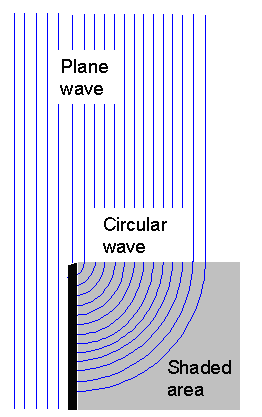The blue lines show the maxima (or minima; whatever you prefer) of the amplitude of a traveling wave at some point in time t0. The distance between the lines is thus the wavelength l Somewhat later, the whole system of lines would have moved somewhat to the right (the propagation direction here). The distance of one wavelength is covered after a time tl = l/v; v is the propagation speed. The time tl is the inverse frequency n Thus we have v = n · l = c for light.The consequences are clear: The resolution dmin of a lens with the numerical aperture NA, i.e. its capability to image two points at a distance dmin separately and not as some blur, is wave-length limited and given by
dmin  »  l
2NAIt's easy to see in a "hand-waving" manner why the numerical aperture comes in. Imagine some lens and reduce its numerical aperture by putting a real opaque aperture with a hole in front of it. The aperture edges will induce a blur that get's worse the smaller the hole and therefore NA. Your resolution goes down with decreasing NA.On the other hand, your lens aberrations become worse with increasing NA. The resulting conflict for optimized optical apparatus is clear and encompasses a lot of intricate and very advanced topics in optics, e.g. how to make structures on microelectronic chips with lateral dimensions around 30 nm < l with optical lithography.Now we consider step 2: two waves can interfere with one another. The principle as shown below is clear.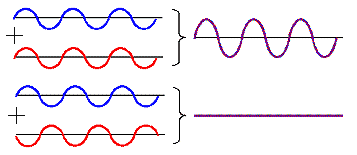For a phase difference = 0 we have constructive interference; the amplitudes are doubled. For a phase difference = 1800 = p  destructive interference "cancels" the wave; the amplitude is zero.The apparent paradox of how you can get nothing from something (where are the two single waves and the energy they carry now?) is not trivial to solve; for details see the link.The paradigmatic experiment for showing interference effects is, of course, the double slit experiment. If you consider that for electron waves, and in particular just for one electron (or photon), you are smack in the middle of quantum theory.In the wave picture the two spherical (or here cylindrical) waves emanating from the two slits interfere to give the pattern shown below. There is no problem at all.In the photon picture, a photon (or electron) passing through the two slits interferes with itself. This boggles the mind quite a bit but the result is the same: You get the interference pattern as shown, with pronounced minima and maxima of the intensity, which now correspond to the probability of detecting the particle.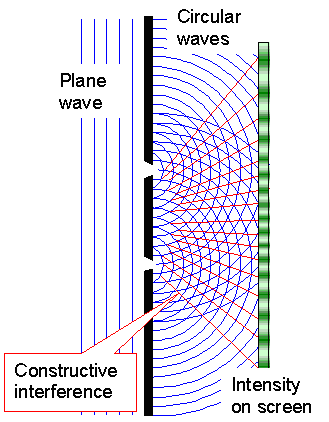Whenever we look at non-trivial optics, we need to consider interference effects. In the real world (as opposed to the ideal world shown in the pictures above), we need to consider the fact that our waves are almost never mono-chromatic (all have the same wavelength) and coherent (all have the same phase) plane waves extending into infinity in every direction.The exception is, of course, the typical Laser beam, where we have an (almost) mono-chromatic and (almost) coherent beam. However, a "Laser beam" is typically "thin" and doesn't extend in all directions. So it is not a simple plane wave!A first important conclusion can be arrived at.If we look at an ensemble of waves with the same wavelength, or better: with the same wave vector k = 2p/l since it contains in addition to the wavelength also the direction of propagation (that's why it's a vector), we note that:
 An ensemble of sufficiently many waves with the same ±k and random phases interferes to exactly zero (plus some noise)"Random phases" means that all phases are equally probable. The proof of the theorem is easy: If one of the many waves has a phase a, there will be some other wave with the phase a - the two will cancel. A visual proof constructed in a different context (that should be familiar) but fits just as well here is shown in the link.From this you realize immediately that inside some hollow tube of length L that reflects waves at either end, only waves with l = 2L/m; m = 1, 2, 3,... can exist.Waves not meeting this criterion will, upon reflection at the end of the tube, produce a phase-shifted wave with some new phase, twice this phase upon the second reflection and so on. Pretty soon you have waves with random phases in there and—see above.What that also means is: We now have also all musical instruments covered, in fact everything where the term "resonator" comes up. This includes also the free electron gas and to some extent electrons in a periodic potential as well as the Bragg condition for diffraction of waves at crystals. The list goes on. Quantum theory deals with wave functions y after all, and the big difference to classical physics comes from the simple fact that you let your wave functions interfere before you take the square, producing in terms of probabilities the classical equivalent of a particle.

Standing WavesIf we look at simple waves propagating in just one direction, i.e. at a one-dimensional problem like sound waves inside an organ pipe, we quickly get the concept of a standing wave, the superposition of two plane waves with everything equal except the sign of the wave vector k.First let's look at the pictures and relations below; they are only meant to refresh your memory

"Running" Plane Wave Standing Wave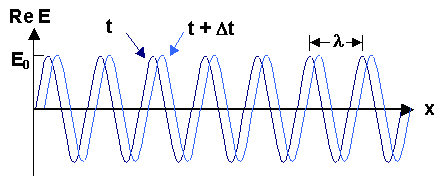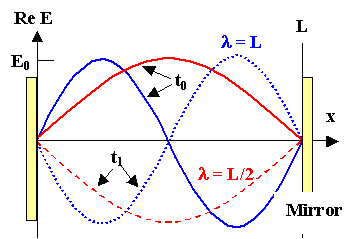E(r,t)   = E0 · exp{i(krwt)}

Re E   = E0 · cos{2p/lwt}
E(r,t)   = E0 · exp{i(krwt)} ± E0 · exp{i(krwt)}

Re E   = 2E0 · cos(2p/l) · cos(wt)

l  = 2L/m; m = 1, 2, 3,..; (a "quantum" number)
L  =  resonator lengthThe pictures and equations are true for acoustic waves, light waves or electron "waves" - just for any wave. You should know standing waves from acoustics - it's the base of any musical instrument, after all, and you hear them all the time.How about standing light waves? You ever seen some?No? So put two mirrors at some distance L in the cm range and admit some light. Are you now going to see a standing light wave between the mirrors, as you should expect from all of the above? No you don't - for several reasons:
1. The coherence length lcoh  of normal light is too short. Normal light is not a infinitely extended plane wave but has some finite "length" that is far below cm. You're essentially missing the extended waves between the mirrors that are superimposed and thus you can't have a standing wave. Organ pipes that are 500 m long don't work either.
2. Fine, so let's use coherent light, however made. OK - you will get standing waves now but you won't notice. The minimal difference in wavelength between two allowed standing waves is Dl = [2L/m – 2L/(m + 1); for large m this simplifies to Dl » 2L/m2. Since l is in the µm region, and L in the cm region, m is around 10.000 and Dl » 10–4 l. In other words, the allowed wavelengths of the standing waves are so close to each other that pretty much all light wavelengths can live inside your resonator. You won't notice a difference to an arbitrary spectrum. Our lecture room here, even so it is a resonator for acoustic waves in principle, doesn't appear to produce nice musical tones because it is simply too large for acoustic wave lengths.
3. OK, so let's make a resonator - two mirrors once more - but only separated by a distance of a few µm. Now you did it. You could have distinct standing light waves in there. But what do you expect to see now? Think!Why do you hear the standing acoustic waves in an organ pipe or in any (classical) musical instrument? Because some of the wave leaks out, eventually hitting your ear. The tone (= pressure amplitude inside the pipe) then would soon be gone if one wouldn't keep feeding acoustic waves into the resonator (by blowing into the organ pipe, for example). Same here. Some light must leak out so you can see it. If the leaking (and the feeding light into the resonator) is done in a certain way, we call the resulting instrument a Laser. We'll get back to this.The long and short is: Yes, interference effects and standing waves are of supreme importance for modern optics and you should refresh you memory about the basics of that if necessary.The following picture shows standing light waves very graphically. We are looking at the unexposed section of a photo resist from microelectronics. The part that was exposed to light has been etched off. The ripples on the left-over resist (= light sensitive polymer) correspond to the extrema of the amplitude of a standing light wave.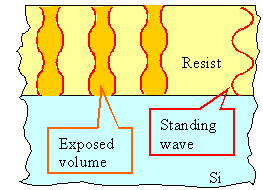The principle of what is happening.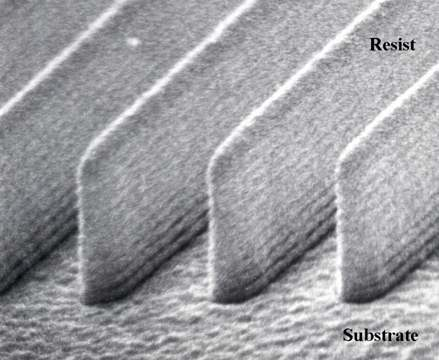The surface of the resist and the surface of the substrate were rather flat and only a few wavelengths apart. When light (monochromatic and rather coherent) was fed to the system, a standing wave developed inside the resist. While you wouldn't have seen anything special, the light intensity "seen" by the resist varied periodically with depth, and that's why the light-induced chemical changes that allow to etch out the exposed part, leave "ripples" on the side walls.If this is all gobbledegook to you, you need to look up "lithography" within the context of semiconductor technologies.
 Questionaire Multiple Choice questions to 5.1.1 - 5.1.3